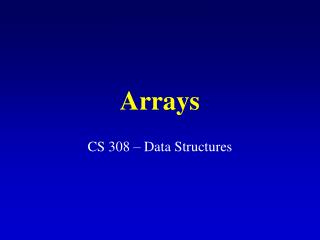Download PresentationArraysArrays - PowerPoint PPT Presentation

Download PresentationArrays
An Image/Link below is provided (as is) to download presentation

Download Policy: Content on the Website is provided to you AS IS for your information and personal use and may not be sold / licensed / shared on other websites without getting consent from its author. While downloading, if for some reason you are not able to download a presentation, the publisher may have deleted the file from their server.

- - - - - - - - - - - - - - - - - - - - - - - - - - - E N D - - - - - - - - - - - - - - - - - - - - - - - - - - -
Presentation Transcript

1. Arrays CS 308 – Data Structures

2. One-Dimensional Arrays • A list of values with the same data type that are stored using a single group name (array name). • General array declaration statement:  data-typearray-name[number-of-items]; • The number-of-items must be specified before declaring the array. const int SIZE = 100; float arr[SIZE];

3. One-Dimensional Arrays (cont.) • Individual elements of the array can be accessed by specifying the name of the array and the element's index: arr • Warning: indices assume values from 0 to number-of-items -1!!

4. One-Dimensional Arrays (cont.) The array name arridentifies the starting location of the array element 3 Skip over 3 elements to get the starting location of element 3 Start here

5. 1D Array Initialization • Arrays can be initialized during their declaration int arr = {98, 87, 92, 79, 85}; int arr = {98, 87} - what happens in this case?? • What is the difference between the following two declarations ?  char codes[] = {'s', 'a', 'm', 'p', 'l', 'e'}; char codes[] = "sample";

6. Two-dimensional Arrays • A two-dimensional array consists of both rows and columns of elements. • General array declaration statement:  data-typearray-name[number-of-rows][number-of-columns];

7. Two-dimensional Arrays (cont.) • The number-of-rows and number-of-columns must be specified before declaring the array. const int ROWS = 100; const int COLS = 50; float arr2D[ROWS][COLS]; • Individual elements of the array can be accessed by specifying the name of the array and the element's row, column indices. arr2D

8. 2D Array Initialization • Arrays can be initialized during their declaration  int arr2D = { {98, 87, 92}, {79, 85, 19}, {32, 18, 2} }; • The compiler fills the array row by row (elements are stored in the memory in the same order).

9. 1D Arrays as Arguments • Individual array elements are passed to a function in the same manner as other variables. max = find_max(arr, arr); • To pass the whole array to a function, you need to specify the name of the array only!!

10. #include <iostream.h> float find_average(int [], int); void main() { const numElems = 5; int arr[numElems] = {2, 18, 1, 27, 16}; cout << "The average is " << find_average(arr, numElems) << endl; } float find_average(int vals[], int n) { int i; float avg; avg=0.0; for(i=0; i<n; i++) avg += vals[i]; avg = avg/n; return avg; }

11. 1D Arrays as Arguments (cont.) • Important: this is essentially "call by reference": • The name of the array arr stores the address of the first element of the array arr (i.e., &arr). • Every other element of the array can be accessed by using its index as an offset from the first element. The starting address of arr array is &arr. This is passed to the function find_average()

12. 2D Arrays as Arguments • Individual array elements are passed to a function in the same manner as other variables.  max = find_max(arr2D, arr2D); • To pass the whole array to a function, you need to specify the name of the array only!! • The number of columns must be specified in the function prototype and function header.

13. #include <iostream.h> float find_average(int [], int, int); void main() { const numRows = 2; const numCols = 2; int arr2D[numRows][numCols] = {2, 18, 1, 27}; float average; average = find_average(arr2D, numRows, numCols); cout << "The average is " << average << endl; }

14. float find_average(int vals[], int n, int m) { int i,j; float avg; avg=0.0; for(i=0; i<n; i++) for(j=0; j<m; j++) avg += vals[i][j]; avg = avg/(n*m); return avg; }

15. 2D Arrays as Arguments (cont.) • Important: this is essentially "call by reference": • The name of the array arr2D stores the address of arr2D (i.e., &arr2D) • arr2D stores the address of the first element of the array arr2D (&arr2D) • Every other element of the array can be accessed by using its indices as an offset from the first element.